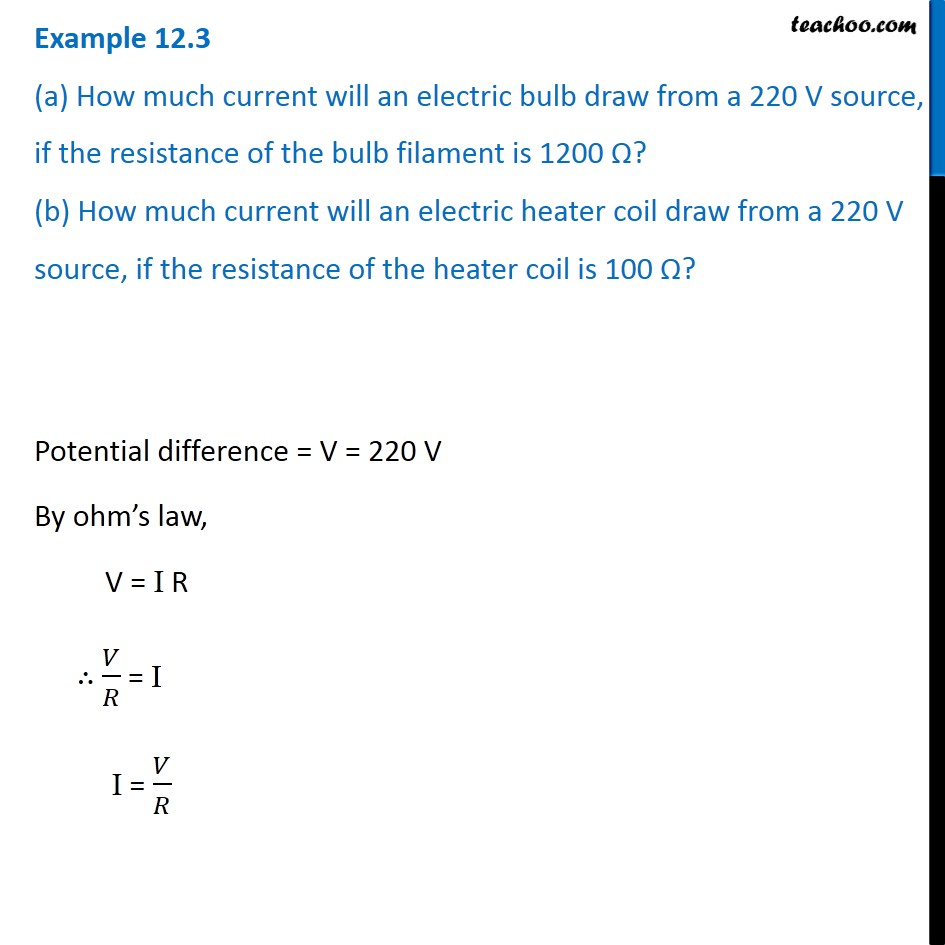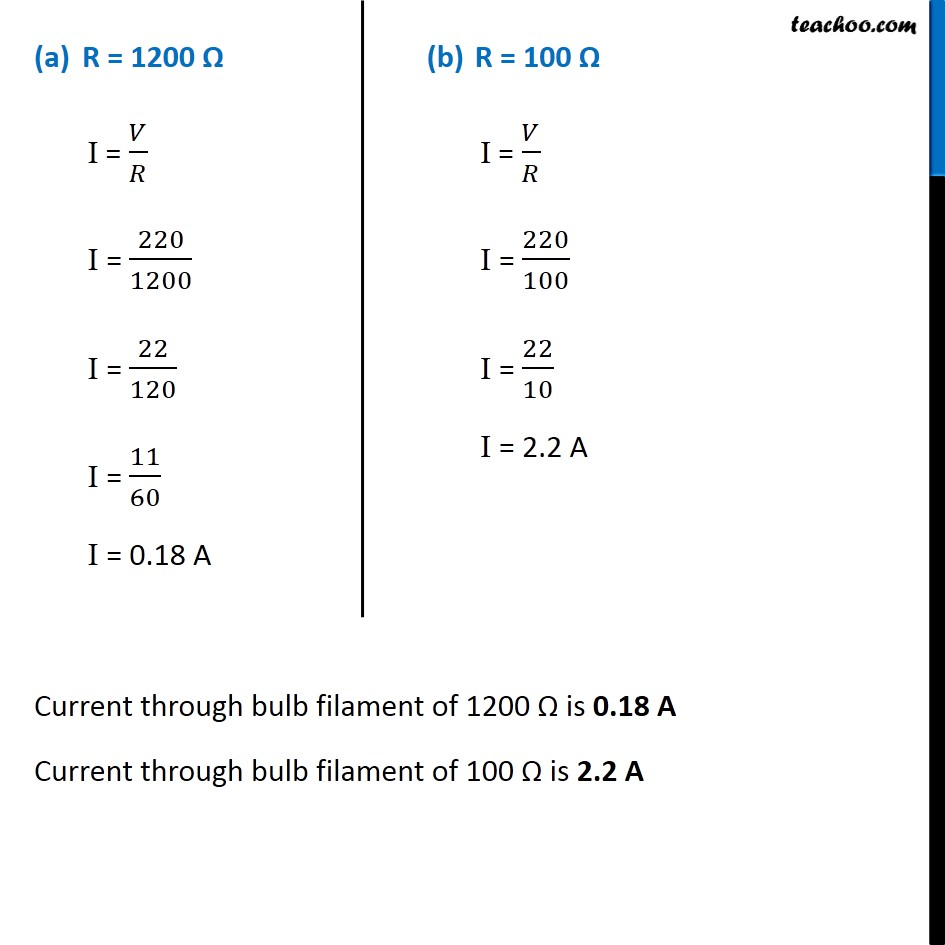Examples from NCERT Book

Class 10
Chapter 12 Class 10 - Electricity

## (a) How much current will an electric bulb draw from a 220 V source, if the resistance of the bulb filament is 1200 Ω? (b) How much current will an electric heater coil draw from a 220 V source, if the resistance of the heater coil is 100 Ω?Learn in your speed, with individual attention - Teachoo Maths 1-on-1 Class

### Transcript

Example 12.3 (a) How much current will an electric bulb draw from a 220 V source, if the resistance of the bulb filament is 1200 Ω? (b) How much current will an electric heater coil draw from a 220 V source, if the resistance of the heater coil is 100 Ω? Potential difference = V = 220 V By ohm’s law, V = I R ∴ 𝑉/𝑅 = I I = 𝑉/𝑅 R = 1200 Ω I = 𝑉/𝑅 I = 220/1200 I = 22/120 I = 11/60 I = 0.18 A R = 100 Ω I = 𝑉/𝑅 I = 220/100 I = 22/10 I = 2.2 A Current through bulb filament of 1200 Ω is 0.18 A Current through bulb filament of 100 Ω is 2.2 A